[an error occurred while processing this directive] [an error occurred while processing this directive]### Question of the Day

On a blank piece of paper (typing paper, no lines) fairly carefully draw the ideal shape that comes to mind when you hear the word “rectangle”. Now measure rectangle and compute the ratio of the longer side (numerator) to the shorter side (denominator). List the ratios from the class.### Key Concepts

1. The Golden Rectangle has some pleasing geometric properties related to the numerical properties of the proportion of its sides, the Golden Ratio.
2. The Golden Ratio, an irrational number appears in a surprising number of mathematical areas.### Vocabulary

1. Golden Ratio: The number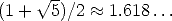which is the solution to the equation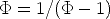2. Golden Rectangle: A rectangle such that the ratio of the longer side tot he shorter side is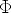.### Mathematical Ideas

#### Construction of the Golden Rectangle

Starting with a square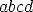, find the midpoint of the side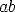. Extend the side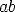. Drop the arc of a circle with center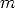and radius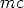to find the pointon the extension of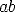. Now erect a perpendicular toat, and also extend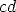to meet the perpendicular at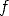. The resulting rectangleis a Golden Rectangle.

#### The Golden Rectangle in Art and Architecture

Some people say that the proportions of the Parthenon in Athens are the proportions of a Golden Rectangle. What do you think? Somme people say that the Golden Rectangle appears many other places in art, including the Mona Lisa, The Last Supper, and early twentieth-century art by Suerat and Mondrian. What do you think?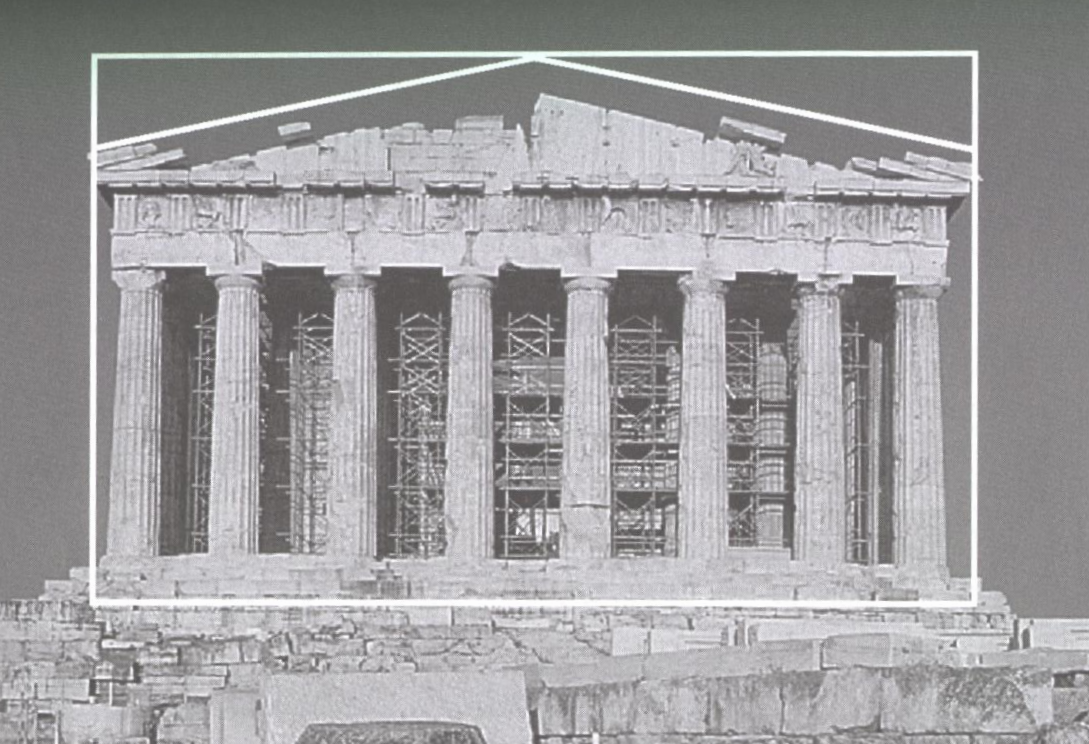This section is adapted from: Instructor Resources and Adjunct Guide for the second edition of The Heart of Mathematics by E. Burger, M. Starbird, and D. Bergstrand.

### Sample Worked Problems

#### Page 244, Problem 4, Same Solution

Why does the equation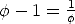have the same solution as the equationAs a solution, suppose that the numberis the solution, and then multiply both sides of the first equation to obtain: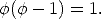Then divide both sides of the equation by the value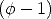to see thatThe result is that the value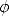satisfies the second equation too!

#### Page 247, Problem 18, Do we get gold this time?

Since we are only interested in proportions or ratios, we can set some convenient length to be 1 and measure all other lengths in terms of this standard. I will take the length of the vertical side of the original (blue in the figure in the book) rectangle. Then the length of the longer horizontal side is 2. The sidelength from the midpoint of the longer horizontal side is 1. Then the radius of the circle which swings to extend the rectangle (by the Pythagorean Theorem) satisfies r2 = 12 + 12 = 2. The radius isand the length of the extended side is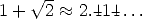. The ratio of the long side to the short side is then 1 +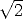and this is not a Golden Rectangle.

#### Page 248, Problem 21, Going platinum

Again, since we are concerned with ratios or proportions, we can choose a convenient side length to be our standard length. Let the sidelength of the vertical side of the rectangle be 1, that is the height of the rectangle is 1. Let the sidelength of the base be b and assume that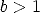as shown, so that the largest square is removed from the base as shown. Then removing the square, we are left with a rectangle of dimensions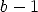and 1. Then the desired ratio isBut this is an impossible equation for lengths, since it implies b = -1. So that means b < 1 so the initial rectangle s tall and thin. (Now draw your own diagram to help you visualize the problem solution.) Then removing the square, we obtainand so solving for b, b = 1/2. This is not a Golden Rectangle, it is a rectangle twice as high as it is wide.